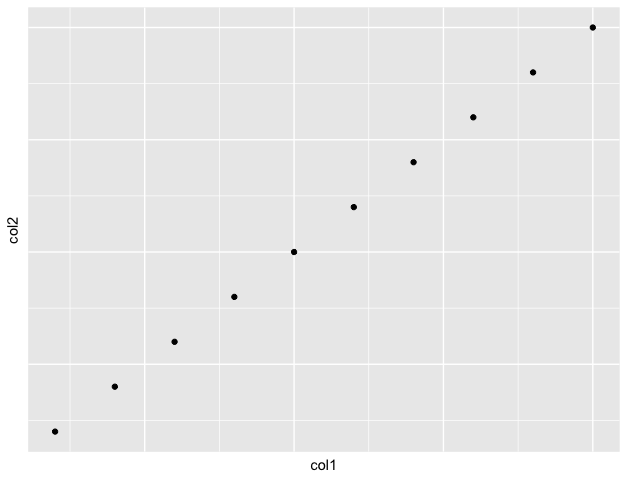# Remove Axis Labels and Ticks in ggplot2 Plot in R

• Last Updated : 24 Oct, 2021

In this article, we will discuss how to remove axis labels and ticks in ggplot2 in R Programming Language.

The axes labels and ticks can be removed in ggplot using the theme() method. This method is basically used to modify the non-data components of the made plot. It gives the plot a good graphical customized look. The theme() method is used to work with the labels, ticks, and text of the plot made. The labels and ticks are aligned to the element_blank() method in order to remove them.

Syntax :

`theme(axis.text.x = , axis.ticks.x = , axis.text.y = , axis.ticks.y = )`

Arguments :

• axis.text.x , axis.text.y =  tick labels along axes
• axis.ticks.x, axis.ticks.y  = tick labels along axes

## R

 `# creating data frame ` `col1 = ``c``(1: 10) ` `col2 = ``c``(11: 20) ` ` `  `print``(``"X coordinates"``) ` `print``(col1) ` ` `  `print``(``"Y coordinates"``) ` `print``(col2) ` ` `  `# plotting the data frame ` `graph < - ``ggplot``(df, ``aes``(x=col1, y=col2)) + ` `geom_point``() ` ` `  `# removing axes labels and ticks ` `graph + ` `theme``(axis.text.x=``element_blank``(), ` `      ``axis.ticks.x=``element_blank``(), ` `      ``axis.text.y=``element_blank``(), ` `      ``axis.ticks.y=``element_blank``()) ` ` `  `# printing the graph ` `print``(graph) `

Output

``` "X coordinates"
  1  2  3  4  5  6  7  8  9 10
 "Y coordinates"
 11 12 13 14 15 16 17 18 19 20```Explanation: The x and y axes markings and tick marks have been removed from the graph by setting them to the element_blank() method.

My Personal Notes arrow_drop_up
Recommended Articles
Page :